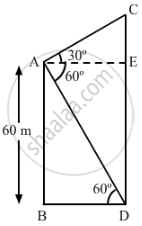Share

# The angles of elevation and depression of the top and the bottom of a tower from the top of a building, 60 m high, are 30° and 60° respectively. Find the difference between the heights of the building and the tower and the distance between them. - CBSE Class 10 - Mathematics

#### Question

The angles of elevation and depression of the top and the bottom of a tower from the top of a building, 60 m high, are 30° and 60° respectively. Find the difference between the heights of the building and the tower and the distance between them.

#### Solution

Let AB be the building and CD be the tower.In right ∆ABD:

(AB)/(BD)=tan 60^@

⇒ 60/(BD)=sqrt3

⇒ BD=60/sqrt3

⇒ BD=20sqrt3

In right ∆ACE:

(CE)/(AE)=tan 30^@

⇒ (CE)/(BD)=1/sqrt3 (∵AE=BD)

⇒CE=(20sqrt3)/sqrt3=20

Height of the tower = CE + ED = CE + AB = 20 m + 60 m = 80 m

Difference between the heights of the tower and the building = 80 m − 60 m = 20 m

Distance between the tower and the building = BD = 20sqrt3

Is there an error in this question or solution?

#### Video TutorialsVIEW ALL 

Solution The angles of elevation and depression of the top and the bottom of a tower from the top of a building, 60 m high, are 30° and 60° respectively. Find the difference between the heights of the building and the tower and the distance between them. Concept: Heights and Distances.
S